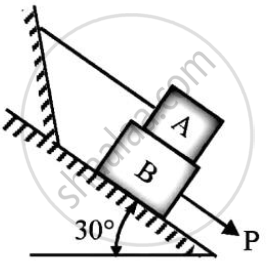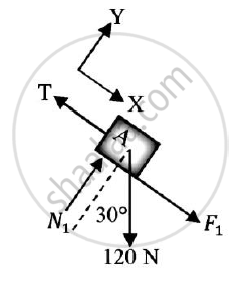# Refer to Figure.If the Co-efficient of Friction is 0.60 for All Contact Surfaces and θ = 30o,What Force P Applied to the Block B Acting Down and Parallel to the Incline Will Start Motion and What Will - Engineering Mechanics

Refer to figure.If the co-efficient of friction is 0.60 for all contact surfaces and θ = 30o,what force P applied to the block B acting down and parallel to the incline will start motion and what will be the tension in the cord parallel to inclined plane attached to A.
Take WA=120 N and WB=200 N.#### Solution

Given : : μ=0.6
θ = 30°
WA = 120 N
WB = 200 N
To find : Force P
Solution :
F1 = μN1 = 0.6N1 ………(1)
F2 = μN2 = 0.6N2 ……….(2)
Consider FBD of block AThe block is considered to be in equilibrium
Applying conditions of equilibrium
ΣFy = 0
N1 - 120cos30 = 0
N1 = 103.923 N ……….(3)
From (1)
F1 = 0.6 x 103.923
= 62.3538 N
Applying conditions of equilibrium
ΣFx = 0
F1 + 120sin30 – T = 0
T = 122.3538 N
Consider FBD of block B
Applying conditions of equilibrium
ΣFy = 0
N2 - N1 - 200cos30 = 0
F2 = 0.6 x 277.1281
= 166.2769 N               From (2)
Applying conditions of equilibrium
ΣFx = 0
P - F1 - F2 + 200sin30 = 0
P = 128.6307 N
Force required on block B to start the motion is 128.6307 N Tension T in the cord parallel to inclined plane attached to A=122.3538 N

Concept: Condition of equilibrium for concurrent forces
Is there an error in this question or solution?